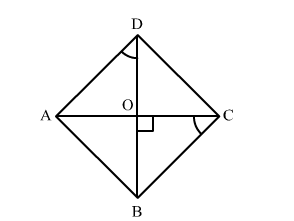# ABCD is a rhombus such that ∠ACB = 50°. Then, ∠ADB = ?

Question:

ABCD is a rhombus such that ∠ACB = 50°. Then, ∠ADB = ?
(a) 40°
(b) 25°
(c) 65°
(d) 130°

Solution:We know that diagonals of rhombus bisect each other at 90°.

Then, in ΔBOC,

90° + 50° + ∠OBC = 180°        (Angle sum property of triangle)

"> ∠OBC = 180° -">- 140°

"> OBC = 40°

But OBC = ADB     (Alternate interior angles)

Thus,